• 如何以最低的经济代价建设这个通信网，是一个网的最小生成树问题。 【基本要求】：（1）利用普里姆算法和克鲁斯卡尔算法求网的最小生成树。 （2）利用堆排序实现选择权值最小的边。 （3）输出生成树中各条边以及他们...c++
• ## 数据结构--最小生成树详解

万次阅读 多人点赞 2017-03-03 19:23:28
Time:2017/3/11、什么是最小生成树现在假设有一个很实际的问题：我们要在n个城市中建立一个通信网络，则连通这n个城市需要布置n-1一条通信线路，这个时候我们需要考虑如何在成本最低的情况下建立这个通信网？...
前言
A wise man changes his mind,a fool never.
Name:Willam
Time:2017/3/1

1、什么是最小生成树

现在假设有一个很实际的问题：我们要在n个城市中建立一个通信网络，则连通这n个城市需要布置n-1一条通信线路，这个时候我们需要考虑如何在成本最低的情况下建立这个通信网？
于是我们就可以引入连通图来解决我们遇到的问题，n个城市就是图上的n个顶点，然后，边表示两个城市的通信线路，每条边上的权重就是我们搭建这条线路所需要的成本，所以现在我们有n个顶点的连通网可以建立不同的生成树，每一颗生成树都可以作为一个通信网，当我们构造这个连通网所花的成本最小时，搭建该连通网的生成树，就称为最小生成树。

构造最小生成树有很多算法，但是他们都是利用了最小生成树的同一种性质：MST性质（假设N=(V,{E})是一个连通网，U是顶点集V的一个非空子集，如果（u，v）是一条具有最小权值的边，其中u属于U，v属于V-U，则必定存在一颗包含边（u，v）的最小生成树），下面就介绍两种使用MST性质生成最小生成树的算法：普里姆算法和克鲁斯卡尔算法。

2、普里姆算法—Prim算法

算法思路：
首先就是从图中的一个起点a开始，把a加入U集合，然后，寻找从与a有关联的边中，权重最小的那条边并且该边的终点b在顶点集合：（V-U）中，我们也把b加入到集合U中，并且输出边（a，b）的信息，这样我们的集合U就有：{a,b}，然后，我们寻找与a关联和b关联的边中，权重最小的那条边并且该边的终点在集合：（V-U）中，我们把c加入到集合U中，并且输出对应的那条边的信息，这样我们的集合U就有：{a,b,c}这三个元素了，一次类推，直到所有顶点都加入到了集合U。

下面我们对下面这幅图求其最小生成树：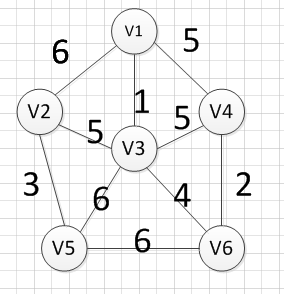假设我们从顶点v1开始，所以我们可以发现（v1,v3）边的权重最小，所以第一个输出的边就是：v1—v3=1: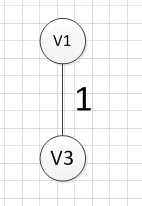然后，我们要从v1和v3作为起点的边中寻找权重最小的边，首先了（v1,v3）已经访问过了，所以我们从其他边中寻找，发现(v3,v6)这条边最小，所以输出边就是：v3—-v6=4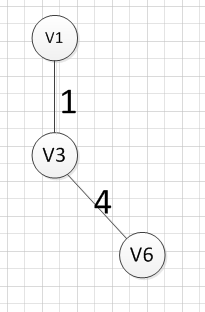然后，我们要从v1、v3、v6这三个点相关联的边中寻找一条权重最小的边，我们可以发现边(v6,v4)权重最小，所以输出边就是：v6—-v4=2.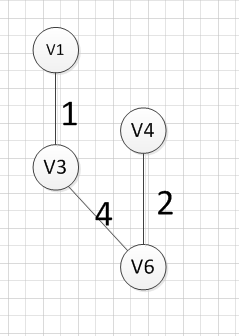然后，我们就从v1、v3、v6、v4这四个顶点相关联的边中寻找权重最小的边，发现边（v3，v2）的权重最小，所以输出边：v3—–v2=5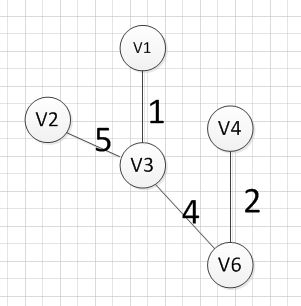然后，我们就从v1、v3、v6、v4，v2这2五个顶点相关联的边中寻找权重最小的边，发现边（v2，v5）的权重最小，所以输出边：v2—–v5=3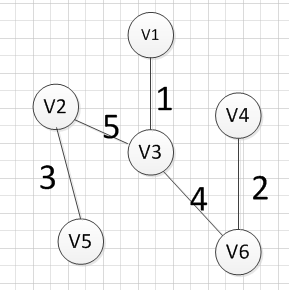最后，我们发现六个点都已经加入到集合U了，我们的最小生成树建立完成。

3、普里姆算法—代码实现

（1）采用的是邻接矩阵的方式存储图，代码如下

#include<iostream>
#include<string>
#include<vector>
using  namespace std;

//首先是使用邻接矩阵完成Prim算法
struct Graph {
int vexnum;  //顶点个数
int edge;   //边的条数
int ** arc; //邻接矩阵
string *information; //记录每个顶点名称
};

//创建图
void createGraph(Graph & g) {
cout << "请输入顶点数：输入边的条数" << endl;
cin >> g.vexnum;
cin >> g.edge;  //输入边的条数

g.information = new string[g.vexnum];
g.arc = new int*[g.vexnum];
int i = 0;

//开辟空间的同时，进行名称的初始化
for (i = 0; i < g.vexnum; i++) {
g.arc[i] = new int[g.vexnum];
g.information[i]="v"+ std::to_string(i+1);//对每个顶点进行命名
for (int k = 0; k < g.vexnum; k++) {
g.arc[i][k] = INT_MAX;          //初始化我们的邻接矩阵
}
}

cout << "请输入每条边之间的顶点编号(顶点编号从1开始),以及该边的权重：" << endl;
for (i = 0; i < g.edge; i++) {
int start;
int end;
cin >> start;   //输入每条边的起点
cin >> end;     //输入每条边的终点
int weight;
cin >> weight;
g.arc[start-1][end-1]=weight;//无向图的边是相反的
g.arc[end-1][start-1] = weight;
}
}

//打印图
void print(Graph g) {
int i;
for (i = 0; i < g.vexnum; i++) {
//cout << g.information[i] << " ";
for (int j = 0; j < g.vexnum; j++) {
if (g.arc[i][j] == INT_MAX)
cout << "∞" << " ";
else
cout << g.arc[i][j] << " ";
}
cout << endl;
}
}

//作为记录边的信息，这些边都是达到end的所有边中，权重最小的那个
struct Assis_array {
int start; //边的终点
int end;  //边的起点
int weight;  //边的权重
};
//进行prim算法实现，使用的邻接矩阵的方法实现。
void Prim(Graph g,int begin) {

//close_edge这个数组记录到达某个顶点的各个边中的权重最大的那个边
Assis_array *close_edge=new Assis_array[g.vexnum];

int j;

//进行close_edge的初始化，更加开始起点进行初始化
for (j = 0; j < g.vexnum; j++) {
if (j != begin - 1) {
close_edge[j].start = begin-1;
close_edge[j].end = j;
close_edge[j].weight = g.arc[begin - 1][j];
}
}
//把起点的close_edge中的值设置为-1，代表已经加入到集合U了
close_edge[begin - 1].weight = -1;
//访问剩下的顶点，并加入依次加入到集合U
for (j = 1; j < g.vexnum; j++) {

int min = INT_MAX;
int k;
int index;
//寻找数组close_edge中权重最小的那个边
for (k = 0; k < g.vexnum; k++) {
if (close_edge[k].weight != -1) {
if (close_edge[k].weight < min) {
min = close_edge[k].weight;
index = k;
}
}
}
//将权重最小的那条边的终点也加入到集合U
close_edge[index].weight = -1;
//输出对应的边的信息
cout << g.information[close_edge[index].start]
<< "-----"
<< g.information[close_edge[index].end]
<< "="
<<g.arc[close_edge[index].start][close_edge[index].end]
<<endl;

//更新我们的close_edge数组。
for (k = 0; k < g.vexnum; k++) {
if (g.arc[close_edge[index].end][k] <close_edge[k].weight) {
close_edge[k].weight = g.arc[close_edge[index].end][k];
close_edge[k].start = close_edge[index].end;
close_edge[k].end = k;
}
}
}
}

int main()
{
Graph g;
createGraph(g);//基本都是无向网图，所以我们只实现了无向网图
print(g);
Prim(g, 1);
system("pause");
return 0;
}

输入：

6 10
1 2 6
1 3 1
1 4 5
2 3 5
2 5 3
3 5 6
3 6 4
4 3 5
4 6 2
5 6 6


输出：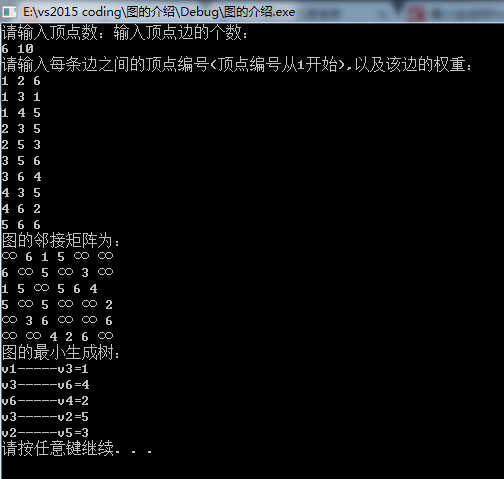时间复杂度的分析：
其中我们建立邻接矩阵需要的时间复杂度为：O(n*n),然后，我们Prim函数中生成最小生成树的时间复杂度为：O(n*n).

（2）采用的是邻接表的方式存储图，代码如下

#include<iostream>
#include<string>
using  namespace std;
//表结点
struct ArcNode {
ArcNode * next;  //指向下一个表结点
int weight;      //边的权重
};
//头结点
struct Vnode {
ArcNode * firstarc;  //第一个和该顶点依附的边 的信息
string data;       //记录该顶点的信息。
};

struct Graph_List {
int vexnum;     //顶点个数
int edge;       //边的条数
Vnode * node;  //顶点表
};

//创建图，是一个重载函数
void createGraph(Graph_List &g) {
cout << "请输入顶点数：输入顶点边的个数：" << endl;
cin >> g.vexnum;
cin >> g.edge;
g.node = new Vnode[g.vexnum];
int i;
for (i = 0; i < g.vexnum; i++) {
g.node[i].data = "v" + std::to_string(i + 1);  //对每个顶点进行命名
g.node[i].firstarc = NULL;//初始化每个顶点的依附表结点
}

cout << "请输入每条边之间的顶点编号(顶点编号从1开始),以及该边的权重：" << endl;
for (i = 0; i < g.edge; i++) {
int start;
int end;
cin >> start;   //输入每条边的起点
cin >> end;     //输入每条边的终点
int weight;
cin >> weight;

ArcNode * next = new ArcNode;
next->next = NULL;
next->weight = weight;
//如果第一个依附的边为空
if (g.node[start - 1].firstarc == NULL) {
g.node[start - 1].firstarc = next;
}
else {
ArcNode * temp; //临时表结点
temp = g.node[start - 1].firstarc;
while (temp->next) {//找到表结点中start-1这个结点的链表的最后一个顶点
temp = temp->next;
}
temp->next = next;  //在该链表的尾部插入一个结点

}
//因为无向图边是双向的
ArcNode * next_2 = new ArcNode;
next_2->weight = weight;
next_2->next = NULL;

//如果第一个依附的边为空
if (g.node[end - 1].firstarc == NULL) {
g.node[end - 1].firstarc = next_2;
}
else {
ArcNode * temp; //临时表结点
temp = g.node[end - 1].firstarc;
while (temp->next) {//找到表结点中start-1这个结点的链表的最后一个顶点
temp = temp->next;
}
temp->next = next_2;  //在该链表的尾部插入一个结点

}

}
}

void print(Graph_List g) {
cout<<"图的邻接表："<<endl;
for (int i = 0; i < g.vexnum; i++) {
cout << g.node[i].data << " ";
ArcNode * next;
next = g.node[i].firstarc;
while (next) {
cout << "("<< g.node[i].data <<","<<g.node[next->adjvex].data<<")="<<next->weight << " ";
next = next->next;
}
cout << "^" << endl;
}
}

作为记录边的信息，这些边都是达到end的所有边中，权重最小的那个
struct Assis_array {
int start; //边的终点
int end;  //边的起点
int weight;  //边的权重
};

void Prim(Graph_List g, int begin) {
cout << "图的最小生成树：" << endl;
//close_edge这个数组记录到达某个顶点的各个边中的权重最大的那个边
Assis_array *close_edge=new Assis_array[g.vexnum];
int j;
for (j = 0; j < g.vexnum; j++) {
close_edge[j].weight = INT_MAX;
}
ArcNode * arc = g.node[begin - 1].firstarc;

while (arc) {
arc = arc->next;
}
//把起点的close_edge中的值设置为-1，代表已经加入到集合U了
close_edge[begin - 1].weight = -1;
//访问剩下的顶点，并加入依次加入到集合U
for (j = 1; j < g.vexnum; j++) {
int min = INT_MAX;
int k;
int index;
//寻找数组close_edge中权重最小的那个边
for (k = 0; k < g.vexnum; k++) {
if (close_edge[k].weight != -1) {
if (close_edge[k].weight < min) {
min = close_edge[k].weight;
index = k;
}
}
}

//输出对应的边的信息
cout << g.node[close_edge[index].start].data
<< "-----"
<< g.node[close_edge[index].end].data
<< "="
<< close_edge[index].weight
<<endl;
//将权重最小的那条边的终点也加入到集合U
close_edge[index].weight = -1;
//更新我们的close_edge数组。
ArcNode * temp = g.node[close_edge[index].end].firstarc;
while (temp) {
}
temp = temp->next;
}
}

}
int main()
{
Graph_List g;
createGraph(g);
print(g);
Prim(g, 1);
system("pause");
return 0;


输入：

6 10
1 2 6
1 3 1
1 4 5
2 3 5
2 5 3
3 5 6
3 6 4
4 3 5
4 6 2
5 6 6


输出：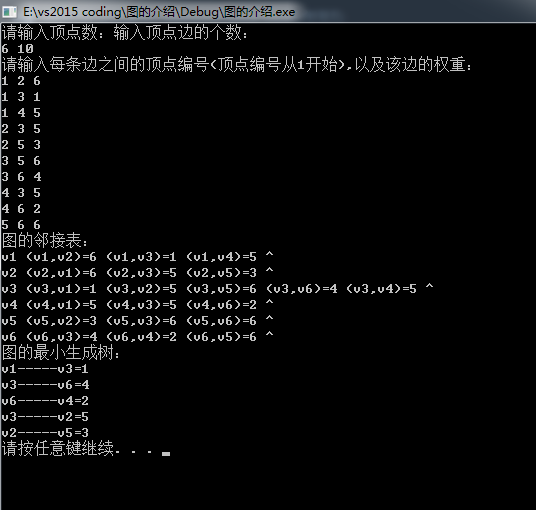时间复杂分析：
在建立图的时候的时间复杂为：O(n+e),在执行Prim算法的时间复杂还是：O(n*n)，总体来说还是邻接表的效率会比较高，因为虽然Prim算法的时间复杂度相同，但是邻接矩阵的那个常系数是比邻接表大的。

另外，Prim算法的时间复杂度都是和边无关的，都是O(n*n)，所以它适合用于边稠密的网建立最小生成树。但是了，我们即将介绍的克鲁斯卡算法恰恰相反，它的时间复杂度为：O(eloge)，其中e为边的条数，因此它相对Prim算法而言，更适用于边稀疏的网。

4、克鲁斯卡算法

算法思路：
（1）将图中的所有边都去掉。
（2）将边按权值从小到大的顺序添加到图中，保证添加的过程中不会形成环
（3）重复上一步直到连接所有顶点，此时就生成了最小生成树。这是一种贪心策略。

这里同样我们给出一个和Prim算法讲解中同样的例子，模拟克鲁斯卡算法生成最小生成树的详细的过程：

首先完整的图如下图：然后，我们需要从这些边中找出权重最小的那条边，可以发现边（v1，v3）这条边的权重是最小的，所以我们输出边：v1—-v3=1然后，我们需要在剩余的边中，再次寻找一条权重最小的边，可以发现边（v4，v6）这条边的权重最小，所以输出边：v4—v6=2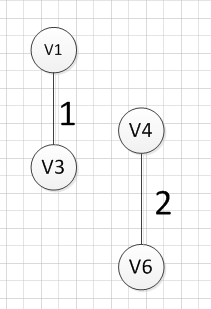然后，我们再次从剩余边中寻找权重最小的边，发现边（v2，v5）的权重最小，所以可以输出边：v2—-v5=3，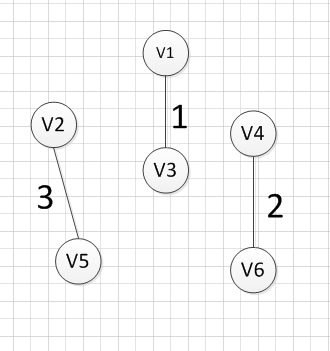然后，我们使用同样的方式找出了权重最小的边：（v3，v6），所以我们输出边：v3—-v6=4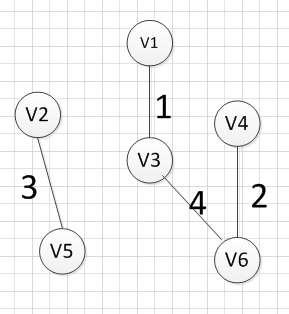好了，现在我们还需要找出最后一条边就可以构造出一颗最小生成树，但是这个时候我们有三个选择：（v1,V4），（v2，v3），（v3，v4）,这三条边的权重都是5，首先我们如果选（v1，v4）的话，得到的图如下：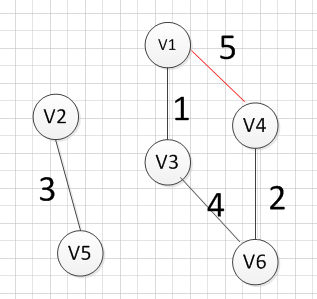我们发现，这肯定是不符合我们算法要求的，因为它出现了一个环，所以我们再使用第二个（v2，v3）试试，得到图形如下：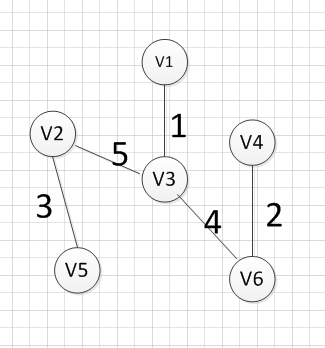我们发现，这个图中没有环出现，而且把所有的顶点都加入到了这颗树上了，所以（v2，v3）就是我们所需要的边，所以最后一个输出的边就是：v2—-v3=5

OK，到这里，我们已经把克鲁斯卡算法过了一遍，下面我们就用具体的代码实现它：

5、克鲁斯卡算法的代码实现

/************************************************************/
/*                程序作者：Willam                          */
/*                程序完成时间：2017/3/3                    */
/*                有任何问题请联系：2930526477@qq.com       */
/************************************************************/
//@尽量写出完美的程序

#include<iostream>
#include<algorithm>
#include<string>
using namespace std;

//检验输入边数和顶点数的值是否有效，可以自己推算为啥：
//顶点数和边数的关系是：((Vexnum*(Vexnum - 1)) / 2) < edge
bool check(int Vexnum,int edge) {
if (Vexnum <= 0 || edge <= 0 || ((Vexnum*(Vexnum - 1)) / 2) < edge)
return false;
return true;
}

//判断我们每次输入的的边的信息是否合法
//顶点从1开始编号
bool check_edge(int Vexnum, int start ,int end, int weight) {
if (start<1 || end<1 || start>Vexnum || end>Vexnum || weight < 0) {
return false;
}
return true;
}

//边集结构，用于保存每条边的信息
typedef struct edge_tag {
bool visit; //判断这条边是否加入到了最小生成树中
int start;   //该边的起点
int end;   //该边的终点
int weight; //该边的权重
}Edge;

//创建一个图，但是图是使用边集结构来保存
void createGraph(Edge * &e,int Vexnum, int edge) {
e = new Edge[edge];//为每条边集开辟空间
int start = 0;
int end = 0;
int weight = 0;

int i = 0;
cout << "输入每条边的起点、终点和权重：" << endl;
while (i != edge)
{
cin >> start >> end >> weight;
while (!check_edge(Vexnum, start, end, weight)) {
cout << "输入的值不合法，请重新输入每条边的起点、终点和权重：" << endl;
cin >> start >> end >> weight;
}
e[i].start = start;
e[i].end = end;
e[i].weight = weight;
e[i].visit = false; //每条边都还没被初始化
++i;

}
}

//我们需要对边集进行排序，排序是按照每条边的权重，从小到大排序。
int cmp(const void*  first, const void * second) {
return ((Edge *)first)->weight - ((Edge *)second)->weight;
}

//好了，我们现在需要做的是通过一定的方式来判断
//如果我们把当前的边加入到生成树中是否会有环出现。
//通过我们之前学习树的知识，我们可以知道如果很多棵树就组成一个森林，而且
//如果同一颗树的两个结点在连上一条边，那么就会出现环，
//所以我们就通过这个方式来判断加入了一个新的边后，是否会产生环，
//开始我们让我们的图的每个顶点都是一颗独立的树，通过不断的组合，把这个森林变
//成来源于同一颗顶点的树
//如果不理解，画个图就明白了，

//首先是找根节点的函数,
//其中parent代表顶点所在子树的根结点
//child代表每个顶点孩子结点的个数
int find_root(int child, int * parent) {

//此时已经找到了该顶点所在树的根节点了
if (parent[child] == child) {
return child;
}
//往前递归，寻找它父亲的所在子树的根结点
parent[child] = find_root(parent[child], parent);
return parent[child];
}

//合并两个子树
bool union_tree(Edge  e, int * parent, int * child) {
//先找出改边所在子树的根节点
int root1;
int root2;
//记住我们顶点从1开始的，所以要减1
root1 = find_root(e.start-1, parent);
root2 = find_root(e.end-1, parent);
//只有两个顶点不在同一颗子树上，才可以把两棵树并未一颗树
if (root1 != root2) {
//小树合并到大树中，看他们的孩子个数
if (child[root1] > child[root2]) {
parent[root2] = root1;
//大树的孩子数量是小树的孩子数量加上
//大树的孩子数量在加上小树根节点自己
child[root1] += child[root2] + 1;
}
else {
parent[root1] = root2;
child[root2] += child[root1] + 1;
}
return true;
}
return false;
}

//克鲁斯卡算法的实现
void Kruskal() {
int Vexnum = 0;
int edge = 0;
cout << "请输入图的顶点数和边数：" << endl;
cin >> Vexnum >> edge;
while (!check(Vexnum, edge)) {
cout << "你输入的图的顶点数和边数不合法，请重新输入：" << endl;
cin >> Vexnum >> edge;
}

//声明一个边集数组
Edge * edge_tag;
//输入每条边的信息
createGraph(edge_tag, Vexnum, edge);

int * parent = new int[Vexnum]; //记录每个顶点所在子树的根节点下标
int * child = new int[Vexnum]; //记录每个顶点为根节点时，其有的孩子节点的个数
int i;
for (i = 0; i < Vexnum; i++) {
parent[i] = i;
child[i] = 0;
}
//对边集数组进行排序，按照权重从小到达排序
qsort(edge_tag, edge, sizeof(Edge), cmp);
int count_vex; //记录输出的边的条数

count_vex = i = 0;
while (i != edge) {
//如果两颗树可以组合在一起，说明该边是生成树的一条边
if (union_tree(edge_tag[i], parent, child)) {
cout << ("v" + std::to_string(edge_tag[i].start))
<< "-----"
<< ("v" + std::to_string(edge_tag[i].end))
<<"="
<< edge_tag[i].weight
<< endl;
edge_tag[i].visit = true;
++count_vex; //生成树的边加1
}
//这里表示所有的边都已经加入成功
if (count_vex == Vexnum - 1) {
break;
}
++i;
}

if (count_vex != Vexnum - 1) {
cout << "此图为非连通图！无法构成最小生成树。" << endl;
}
delete [] edge_tag;
delete [] parent;
delete [] child;
}

int main() {
Kruskal();
system("pause");
return 0;
}

输入：

6 10
1 2 6
1 3 1
1 4 5
2 3 5
2 5 3
3 5 6
3 6 4
4 3 5
4 6 2
5 6 6


输出：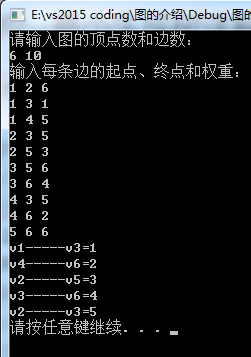输入：

7 9
1 2 20
1 5 1
2 3 6
2 4 4
3 7 2
4 6 12
4 7 8
5 6 15
6 7 10

输出：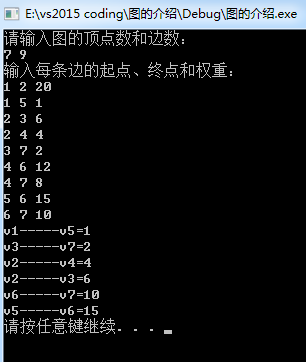展开全文Kruskal Prim
• 数据结构 最小生成树 Kruskal算法 无向连通图的生成树不是唯一的。连通图的一次遍历所经过的边的集合及图中所有顶点的集合就构成了该图的一棵生成树，对连通图的不同遍历，就可能得到不同的生成树，那么，它的所有...
数据结构  最小生成树  Kruskal算法

无向连通图的生成树不是唯一的。连通图的一次遍历所经过的边的集合及图中所有顶点的集合就构成了该图的一棵生成树，对连通图的不同遍历，就可能得到不同的生成树，那么，它的所有生成树中必有一棵边的权值总和最小的生成树，我们称这棵生成树为最小生成树，简称为最小生成树

最小生成树：
具有最小权重且连接到所有结点的树

对于带权无向图G=(V, E),V表示图中的结点，E表示图中的边，最小生成树为集合T, T是以最小代价连接V中所有顶点所用边E的最小集合（就是联通图G中所有结点所需要的边长度总和最小）。 集合T中的边能够形成一颗树，因为每个节点（除根节点）都能向上找到它的一个父节点，这些边加上V就构成图G的一颗最小生成树

安全边：
假设A是某棵最小生成树的子集，下一步我们需要做的就是找出一条边(u,v)，将其加入到A中，使得A∪{(u,v)}也是某棵最小生成树的子集,我们称(u,v)为安全边
切割(S,V-S)：
—对集合V的一个划分，将集合V划分为两个部分S和V-S
横跨切割：
—若边(u,v)的一个节点属于S另一个节点属于V-S，则称(u,v)横跨切割
尊重集合A：
如果A中不存在横跨切割的边，则称该集合尊重集合A

横跨尊重集合A的切割(S,V-S)的一条轻量级边(u,v)为A的一条安全边

Prim算法和Kruskal算法在实现方面的区别：
1、Kruskal算法在生成最小生成树的过程中产生的是森林，
Prim算法在执行过程中始终都是一棵树；
2、Kruskal和Prim实现上的最大区别：
Kruskal不需要搜索每个顶点的邻接节点，
Prim图构建时需要利用邻接链表进行构建，Kruskal不用！
3、Kruskal算法在效率上要比Prim算法快，
Kruskal只需要对权重边做一次排序
而Prim算法则需要做多次排序

Prim算法是依赖于点的算法：
它的基本原理是从当前点寻找一个离自己（集合）最近的点然后把这个点拉到自己家来（距离设为0），同时输出一条边，并且刷新到其他点的路径长度。俗称，刷表。
根据Prim算法的特性可以得知，它很适合于点密集的图。对Prim算法通常采用邻接矩阵的储存结构。这种储存方法空间复杂度N^2，时间复杂度N^2。
对于稍微稀疏一点的图，其实我们更适合采用邻接表的储存方式，可以节省空间，并在一定条件下节省时间。

Kruskal算法是依赖边的算法：
基本原理是将边集数组排序，然后通过维护一个并查集来分清楚并进来的点和没并进来的点，依次按从小到大的顺序遍历边集数组，如果这条边对应的两个顶点不在一个集合内，就输出这条边，并合并这两个点。

根据Kruskal算法的特性可得，在边越少的情况下，Kruskal算法相对Prim算法的优势就越大。同时，因为边集数组便于维护，所以Kruskal在数据维护方面也较为简单，不像邻接表那样复杂。Kruskal算法的速度与点数无关，对于稀疏图可以采用Kruskal算法。

Kruskal算法(克鲁斯卡尔算法)

算法原理：
首先将每个顶点放入其自身的数据集合中。然后，按照权值的升序来选择边。当选择每条边时，判断定义边的顶点是否在不同的数据集中。如果是，将此边插入最小生成树的集合中，同时，将集合中包含每个顶点的联合体取出，如果不是，就移动到下一条边。重复这个过程直到所有的边都探查过

Kruskal在找最小生成树结点之前，需要对所有权重边做从小到大排序。将排序好的权重边依次加入到最小生成树中，如果加入时产生回路就跳过这条边，加入下一条边。当所有结点都加入到最小生成树中之后，就找出了最小生成树

算法描述：
1、新建图G，把图G中所有的边按照权值从小到大排序。(一般使用优先队列)
2、取出最小的边，如果这条边连接的两个节点于图G中不在同一个连通分量中，则添加这条边到图G中.(使用并查集)
3、重复步骤2，直至图G中所有的节点都在同一个连通分量中

性能分析
Kruskal算法，基本上取决于优先队列的选择，以及并查集的实现。
算法效率为 O(ElogE) E为图中的边数

时间复杂度：O(Elog2E) E为图中的边数
//对优先队列和并查集 //查找算法之顺序、二分、二叉搜索树、红黑树 和并查集

import java.io.IOException;
import java.util.Scanner;

public class Kruskal {
private int mEdgNum;        // 边的数量
private char[] mVexs;       // 顶点集合
private int[][] mMatrix;    // 邻接矩阵
private static final int INF = 100;//Integer.MAX_VALUE;   // 最大值

//创建图(用已提供的矩阵)
public Kruskal(char[] vexs, int[][] matrix) {// vexs-- 顶点数组 ,matrix-- 矩阵(数据)
// 初始化"顶点数"和"边数"
int vlen = vexs.length;
// 初始化"顶点"
mVexs = new char[vlen];
for (int i = 0; i < mVexs.length; i++)
mVexs[i] = vexs[i];
// 初始化"边"
mMatrix = new int[vlen][vlen];
for (int i = 0; i < vlen; i++)
for (int j = 0; j < vlen; j++)
mMatrix[i][j] = matrix[i][j];
// 统计"边"
mEdgNum = 0;
for (int i = 0; i < vlen; i++)
for (int j = i+1; j < vlen; j++)
if (mMatrix[i][j]!=INF)
mEdgNum++;
}

//返回ch位置
private int getPosition(char ch) {
for(int i=0; i<mVexs.length; i++)
if(mVexs[i]==ch)
return i;
return -1;
}

// 返回顶点v的第一个邻接顶点的索引，失败则返回-1
private int firstVertex(int v) {
if (v<0 || v>(mVexs.length-1))
return -1;
for (int i = 0; i < mVexs.length; i++)
if (mMatrix[v][i]!=0 && mMatrix[v][i]!=INF)
return i;
return -1;
}

//打印邻接顶点的索引、权重
private void nextVertexs() {
for(int v = 0; v < mVexs.length; v++){
System.out.print("\n结点"+v);
for (int i = 0; i < mVexs.length; i++) {
if (mMatrix[v][i]!=0 && mMatrix[v][i]!=INF)
System.out.print("邻接点"+i+"(权重"+mMatrix[v][i]+")");
}
}
}

//打印矩阵队列图
public void print() {
int i, j, sum;
System.out.printf("结点表:");
for (i=0; i < mVexs.length; i++) {
sum = 0;
for (j=0; j < mVexs.length; j++) {
if (mMatrix[i][j]!=0 && mMatrix[i][j]!=INF) {
sum++;
}
}
System.out.print("\n结点"+i+", 标识"+mVexs[i]+", 共有邻接点"+sum);
}

System.out.print("\n\n");
System.out.printf("邻接矩阵:\n");
for (i = 0; i < mVexs.length; i++) {
for (j = 0; j < mVexs.length; j++)
System.out.printf("%3d ", mMatrix[i][j]);
System.out.printf("\n");
}

nextVertexs();
}

/*
* 克鲁斯卡尔（Kruskal)最小生成树
*/
public void kruskal() {
int index = 0;                      // rets数组的索引
int[] vends = new int[mEdgNum];     // 用于保存"已有最小生成树"中每个顶点在该最小树中的终点。
EData[] rets = new EData[mEdgNum];  // 结果数组，保存kruskal最小生成树的边
EData[] edges;                      // 图对应的所有边

edges = getEdges(); // 获取"图中所有的边"
sortEdges(edges, mEdgNum); // 将边按照"权"的大小进行排序(从小到大)

for (int i=0; i<mEdgNum; i++) {
int p1 = getPosition(edges[i].start);      // 获取第i条边的"起点"的序号
int p2 = getPosition(edges[i].end);        // 获取第i条边的"终点"的序号

int m = getEnd(vends, p1);                 // 获取p1在"已有的最小生成树"中的终点
int n = getEnd(vends, p2);                 // 获取p2在"已有的最小生成树"中的终点
// 如果m!=n，意味着"边i"与"已经添加到最小生成树中的顶点"没有形成环路
if (m != n) {
vends[m] = n;                       // 设置m在"已有的最小生成树"中的终点为n
rets[index++] = edges[i];           // 保存结果
}
}

// 统计并打印"kruskal最小生成树"的信息
int length = 0;
for (int i = 0; i < index; i++)
length += rets[i].weight;

System.out.printf("\nKruskal=%d: ", length);
for (int i = 0; i < index; i++)
System.out.printf("(%c,%c) ", rets[i].start, rets[i].end);
System.out.printf("\n");
}

//获取图中的边
private EData[] getEdges() {
int index=0;
EData[] edges;
edges = new EData[mEdgNum];
for (int i=0; i < mVexs.length; i++) {
for (int j=i+1; j < mVexs.length; j++) {
if (mMatrix[i][j]!=INF) {
edges[index++] = new EData(mVexs[i], mVexs[j], mMatrix[i][j]);
}
}
}
return edges;
}

//对边按照权值大小进行排序(由小到大)
private void sortEdges(EData[] edges, int elen) {
for (int i=0; i<elen; i++) {
for (int j=i+1; j<elen; j++) {
if (edges[i].weight > edges[j].weight) {// 交换"边i"和"边j"
EData tmp = edges[i];
edges[i] = edges[j];
edges[j] = tmp;
}
}
}
}

//获取i的终点
private int getEnd(int[] vends, int i) {
while (vends[i] != 0)
i = vends[i];
return i;
}

// 边的结构体
private static class EData {
char start; // 边的起点
char end;   // 边的终点
int weight; // 边的权重
public EData(char start, char end, int weight) {
this.start = start;
this.end = end;
this.weight = weight;
}
};

public static void main(String[] args) {
char[] vexs = {'A', 'B', 'C', 'D', 'E', 'F', 'G'};
int matrix[][] = {
/*A*//*B*//*C*//*D*//*E*//*F*//*G*/
/*A*/ {   0,  12, INF, INF, INF,  16,  14},
/*B*/ {  12,   0,  10, INF, INF,   7, INF},
/*C*/ { INF,  10,   0,   3,   5,   6, INF},
/*D*/ { INF, INF,   3,   0,   4, INF, INF},
/*E*/ { INF, INF,   5,   4,   0,   2,   8},
/*F*/ {  16,   7,   6, INF,   2,   0,   9},
/*G*/ {  14, INF, INF, INF,   8,   9,   0}};
Kruskal pG;
pG = new Kruskal(vexs, matrix);

pG.print();   // 输出图的有关信息

pG.kruskal();   // Kruskal算法生成最小生成树
}
}

程序输出：

结点表:
结点0, 标识A, 共有邻接点3
结点1, 标识B, 共有邻接点3
结点2, 标识C, 共有邻接点4
结点3, 标识D, 共有邻接点2
结点4, 标识E, 共有邻接点4
结点5, 标识F, 共有邻接点5
结点6, 标识G, 共有邻接点3

邻接矩阵:
0  12 100 100 100  16  14
12   0  10 100 100   7 100
100  10   0   3   5   6 100
100 100   3   0   4 100 100
100 100   5   4   0   2   8
16   7   6 100   2   0   9
14 100 100 100   8   9   0

结点0邻接点1(权重12)邻接点5(权重16)邻接点6(权重14)
结点1邻接点0(权重12)邻接点2(权重10)邻接点5(权重7)
结点2邻接点1(权重10)邻接点3(权重3)邻接点4(权重5)邻接点5(权重6)
结点3邻接点2(权重3)邻接点4(权重4)
结点4邻接点2(权重5)邻接点3(权重4)邻接点5(权重2)邻接点6(权重8)
结点5邻接点0(权重16)邻接点1(权重7)邻接点2(权重6)邻接点4(权重2)邻接点6(权重9)
结点6邻接点0(权重14)邻接点4(权重8)邻接点5(权重9)

Kruskal=36: (E,F) (C,D) (D,E) (B,F) (E,G) (A,B) 
展开全文kruskal
• 这种构造连通网的最小代价生成树称为最小生成树，详见数据结构之图（术语、存储结构、遍历）。 求连通网的最小生成树有两种经典方法：普里姆（Prime）算法和克鲁斯卡尔（Kruskal）算法。 1、Prime算法 (1)算法描述 ...
最小生成树： 一个连通图的生成树是一个极小连通子图，它含有图中全部顶点，但只有足以构成一棵树的n-1条边。这种构造连通网的最小代价生成树称为最小生成树，详见数据结构之图（术语、存储结构、遍历）。
求连通网的最小生成树有两种经典方法：普里姆（Prime）算法和克鲁斯卡尔（Kruskal）算法。
1、Prime算法
(1)算法描述
假设N=(V,{E})是连通网，TE是N上最小生成树中边的集合。从V中任选一个顶点u0，算法从U={u0}(u0∈V)，TE={}开始，重复执行以下步骤：
在所有u∈U、v∈V-U的边(u, v)∈E中找一条代价最小的边(u, vi)并入集合TE，同时将vi并入U，直至U=V为止。
此时TE中必有n-1条边，则T={V,{TE}}即为N的最小生成树。
需要用到两个辅助数组：
lowcost[MAX_VEM]：存储从U到V-U的具有最小代价边上的权——lowcost[i] = Min{w(u, vi)|u∈U,vi∈V-U}
(2)实例
给定如下一个无向网：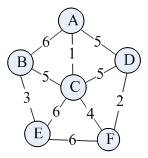根据上述prime算法描述，求其最小生成树的过程如下：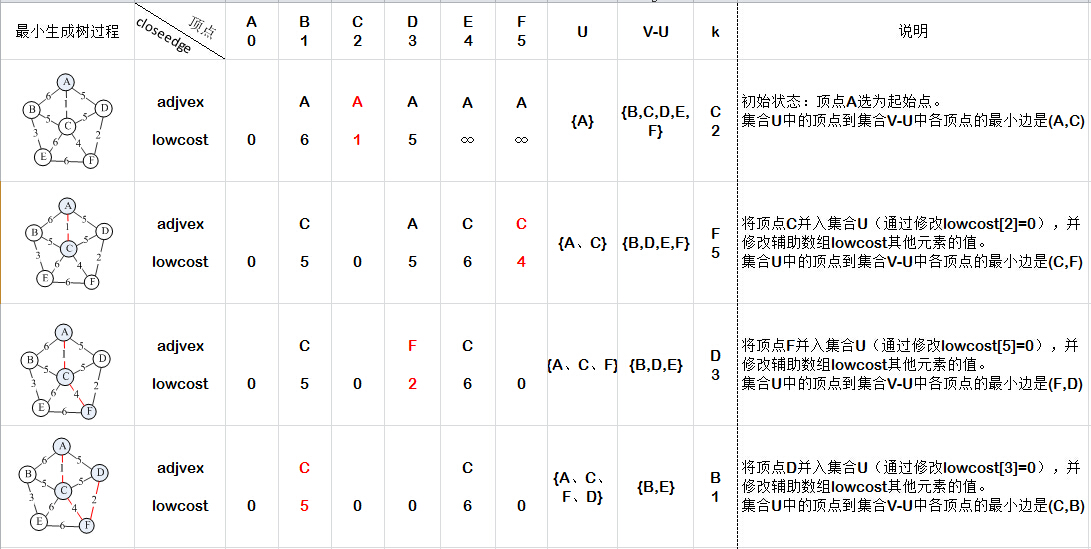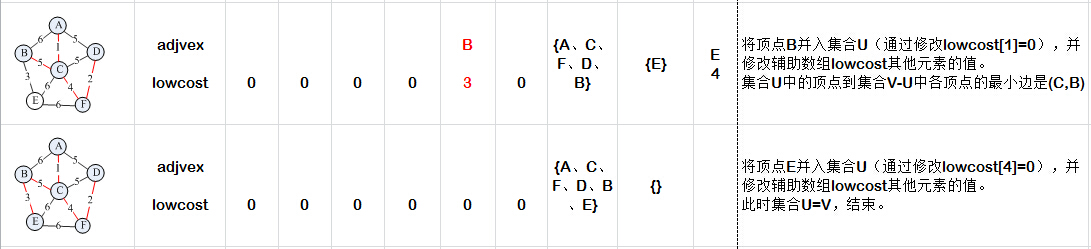最后得到如下最小生成树：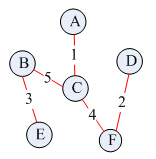(3)、算法代码
//普里姆(Prime)算法
void MiniSpanTree_Prime(Graph *g, VertexType u0)
{
int min, i, j, k, v0;

//adjvex[i] = {u|lowcost[i] = Min{w(u, vi)|u∈U,vi∈V-U}}
int lowcost[MAX_VEX];	//存储从U到V-U的具有最小代价边上的权——lowcost[i] = Min{w(u, vi)|u∈U,vi∈V-U}

v0 = k = LocateVex(g, u0);	//定位开始的顶点u0在顶点数组中的下标号
assert(k != -1);
lowcost[k] = 0;	//初始,U={u}

for (i = 0; i < g->vexNum; i++)
{
if (i != k)	//初始化除下标为k的其余全部顶点
{
lowcost[i] = g->arc[k][i];	//将顶点u与之有关的权值存入数组
}
}

for (i = 0; i < g->vexNum; i++)
{
if (i == v0)
{
continue;
}

min = INFINITY;
for (j = 0, k = 0; j < g->vexNum; j++)
{
if (lowcost[j] != 0 && lowcost[j] < min)   //V-U中的全部顶点
{
min = lowcost[j];
k = j;
}
}

lowcost[k] = 0; //第k顶点并入U集

for (j = 0; j < g->vexNum; j++)
{
if (lowcost[j] != 0 && g->arc[k][j] < lowcost[j])
{
lowcost[j] = g->arc[k][j];	//新顶点并入U后重新选择最小边
}
}
}
putchar('\n');
}
运行截图：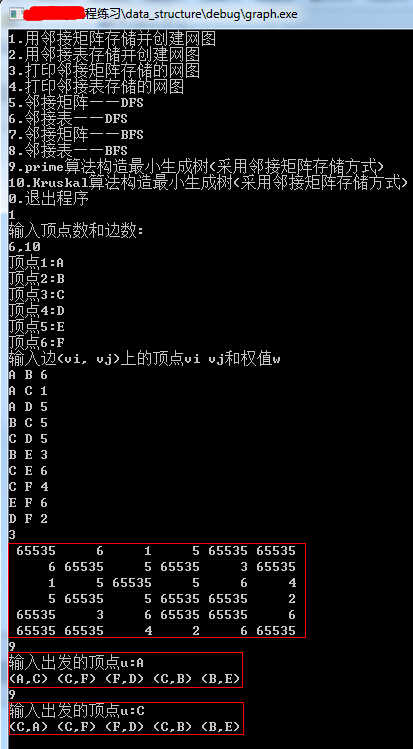分析：邻接矩阵实现的普里姆算法的时间复杂度为O(n^2)，与网中的边数无关，适用于求边稠密的网的最小生成树。

2、Kruskal算法
(1)算法描述
假设连通网N={V,{E}}，令最小生成树的初始状态为只有n个顶点而无边的非连通图T={V,{}}，图中的每个顶点自成一个连通分量。在E中选择代价最小的边，若改边依附的顶点落在T中不同的连通分量上，则将次边加入到T中，否则舍去此边而选择下一条代价i最小的边。以此类推，直到T中所有顶点都在同一个连通分量上为止。
Kruskal算法主要考虑是否会形成环路。在实际的代码编写中，一般采用边集数组这一数据结构：

//边集数组
#define  MAX_EDGE	100	//最大边数
typedef struct
{
int begin;
int end;
EdgeType weight;
}Edge;

我们可以通过程序将邻接矩阵通过程序转化为边集数组，并且对它们的按权值从小到大排序。如下图所示。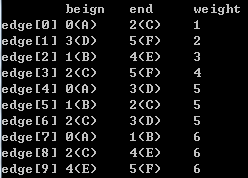（2）算法代码

//将邻接矩阵结构转化成边集
void Convert(Graph *g, Edge edge[])
{
int i, j, k = 0;
for (i = 0; i < g->vexNum; i++)
for (j = i; j < g->vexNum; j++)	 //无向图的邻接矩阵是对称的
if (g->arc[i][j] < INFINITY)
{
edge[k].begin = i;
edge[k].end = j;
edge[k].weight = g->arc[i][j];
k++;
}
#if 0
printf("排序前:\n");
printf("       \tbeign\tend\tweight\n");
for(i = 0; i < k; i++)
{
printf("edge[%d]\t%d(%c)\t%d(%c)\t%d\n", i,
edge[i].begin, g->vexs[edge[i].begin],
edge[i].end, g->vexs[edge[i].end], edge[i].weight);
}
#endif
InsertSort(edge, k);
#if 1
printf("排序后:\n");
printf("       \tbeign\tend\tweight\n");
for(i = 0; i < k; i++)
{
printf("edge[%d]\t%d(%c)\t%d(%c)\t%d\n", i,
edge[i].begin, g->vexs[edge[i].begin],
edge[i].end, g->vexs[edge[i].end], edge[i].weight);
}
#endif
}

//按权值大小对边集数组edge从小至大排序
void InsertSort(Edge edge[], int k)
{
int i, j;
Edge tmp;

for (i = 1; i < k; i++)
{
if (edge[i].weight < edge[i - 1].weight)
{
tmp = edge[i];
for (j = i - 1; edge[j].weight > tmp.weight; j--)
{
edge[j + 1] = edge[j];
}
edge[j + 1] = tmp;
}
}
}

//查找连线顶点的尾部
int Find(int *parent, int f)
{
while(parent[f] > 0)
{
f = parent[f];
}
return f;
}

//克鲁斯卡尔算法实现
void MiniSpanTree_Kruskal(Graph *g)
{
int i, n, m;
Edge edges[MAX_EDGE];    //定义边集数组
int parent[MAX_EDGE];     //定义一数组用来判断边与边是否形成环

//将邻接矩阵转化为边集数组edges并按权值由小到大排序
Convert(g, edges);

for(i = 0; i < g->vexNum; i++)
{
parent[i] = 0;  //初始化数组值为0
}

for(i = 0; i < g->arcNum; i++)          //循环每一条边
{
n = Find(parent, edges[i].begin);
m = Find(parent, edges[i].end);
if(n != m)      //假如n与m不等，说明此边没有与现有生成树形成环路
{
parent[n] = m;  //将此边的结尾顶点放入下为起点的parent数组中
//表示此顶点已经在生成树集合中
//printf("(%d,%d) %d ", edges[i].begin, edges[i].end, edges[i].weight);
printf("(%c,%c) ", g->vexs[edges[i].begin], g->vexs[edges[i].end]);
}
}
putchar('\n');
}

运行截图：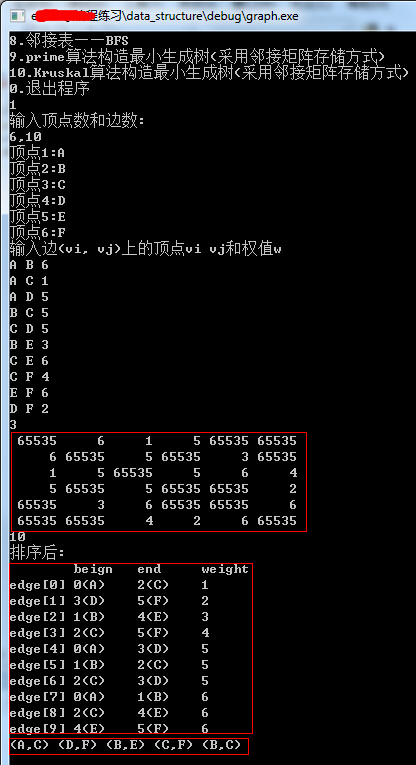不考虑邻接矩阵或邻接表转化为边集数组的时间开销，克鲁斯卡尔算法的Find函数由边数e决定，时间复杂度为O(loge)，而外面有一个for循环e次，所以克鲁斯卡尔算法的时间复杂度为O(eloge)，相对prime算法而言，适合于求边稀疏的网的最小生成树。

参考：这篇文章的url提示不对呀 “** 文章包含被禁用的url，无法保存和发布。” ，没法给出参考链接了
数据结构（C语言版）
完整的测试代码：http://download.csdn.net/detail/u013071074/7467561
展开全文Kruskal
• 其实应用最小生成树能够很好的解决这类问题。即给定一幅加权无向图，找到它的一颗最小生成树。此类算法主要应用是电路元器件的设计，航空航线规划，电站电力分配，图像分析等领域。 最小生成树   图的生成树是它的...
Python数据结构最小生成树前言最小生成树最小生成树的经典算法Python实现理论证明切分定理贪心算法Prim算法知识补充切分的定义系列补充
前言
最近收到电力类专业同学的求助，希望能用一个算法使得从变电站至所有负载的总路线最短。当然，如果要添加特殊要求，你可以通过我的邮箱707101557@qq.com联系我。其实应用最小生成树能够很好的解决这类问题。即给定一幅加权无向图，找到它的一颗最小生成树。此类算法主要应用是电路元器件的设计，航空航线规划，电站电力分配，图像分析等领域。
如果只是简单使用，建议你直接带走本文所提供的代码，这份代码是易于使用的。如果你需要深入了解，我也在此新增了理论证明部分。
最小生成树
图的生成树是它的一棵含有其所有顶点的无环连通子图。一幅加权图的最小生成树(MST)是它的一棵权值(图中所有边的权值之和)最小的生成树。
最小生成树的经典算法
找最小生成树的两种经典算法分别是Prim算法和Kruskal算法。
Prim算法每一步都会为一棵生长中的树加一条边。一开始这看树只有一个顶点，然后会向它添加V-1条边，每次总是将下一条连接树中的顶点与不在树中的顶点且权重最小的边加入树中。
笔者只是编写了该算法Python版本，读者可以通过VisuAlgo查看该算法的动态演示过程。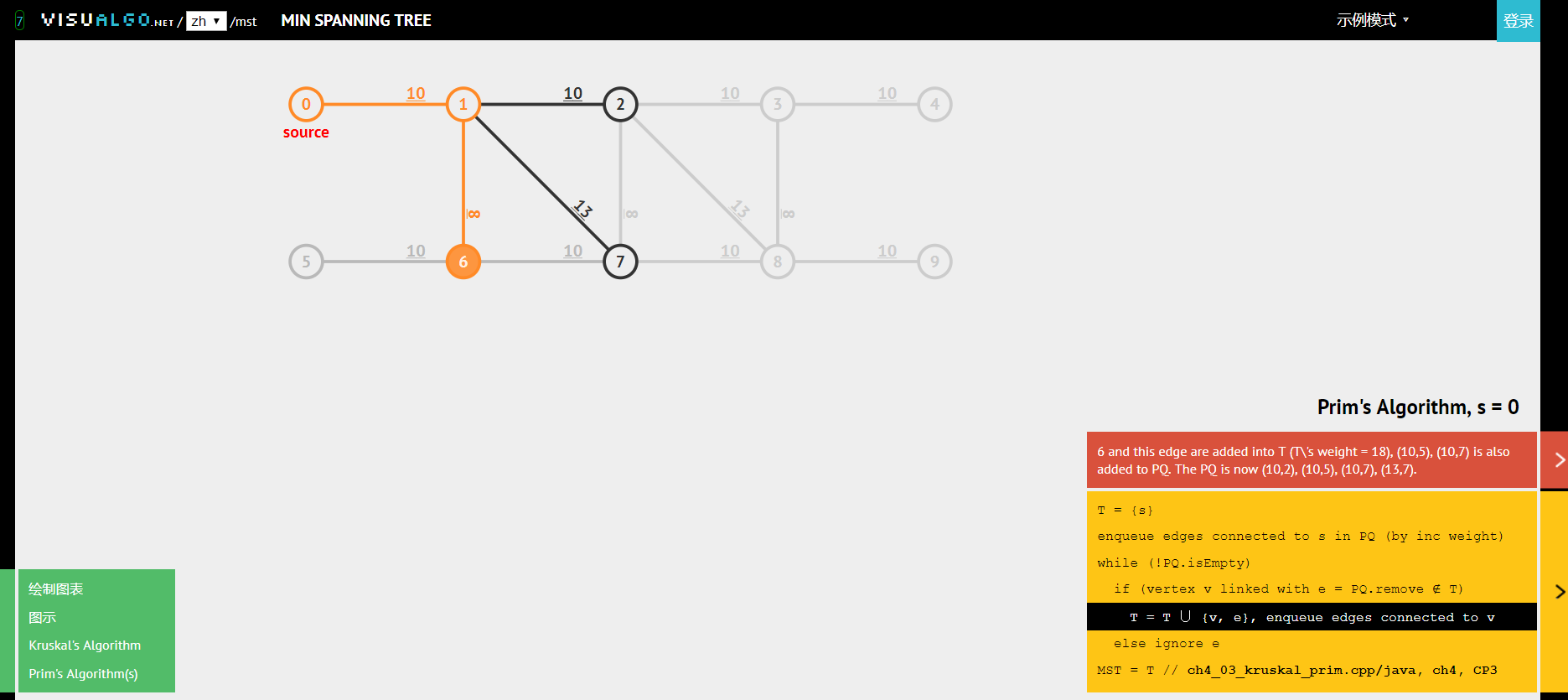Python实现
#file @mst.py
#date @2019/10/26
#Program to find the Minimum Spanning Tree using Prim's Algorithm and Heap Data Structure

Maxint = 2**31-1
#Implementation of Heap
class Heap:

s = 0
a = []
p = {}
key = {}

def __init__(self):
self.s = -1

def heapify_up(self, i):
while i>0:
j = i//2
if self.key[self.a[i]] < self.key[self.a[j]]:
temp = self.a[i]
self.a[i] = self.a[j]
self.a[j] = temp
self.p[self.a[i]] = i
self.p[self.a[j]] = j
i = j
else:
break

def heapify_down(self,i):
j=-1
while 2*i <= self.s:
if 2*i == self.s or self.key[self.a[2*i]] <= self.key[self.a[2*i + 1]]:
j = 2*i
else:
j = 2*i + 1
if self.key[self.a[j]] < self.key[self.a[i]]:
temp = self.a[i]
self.a[i] = self.a[j]
self.a[j] = temp
self.p[self.a[i]] = i
self.p[self.a[j]] = j
i = j
else:
break

def decrease_key(self, v, key_value):
self.key[v] = key_value
self.heapify_up(self.p[v])

def extract_min(self):
ret = self.a
self.a=self.a[self.s]
self.p[self.a] = 0
self.s-=1
if self.s>=0:
self.heapify_down(0)
return ret

def insert(self, v, key_value):
self.a.append(v)
self.s +=1
self.p[v] = self.s
self.key[v] = key_value
self.heapify_up(self.s)

def printdata(self):
print("Value of array a: ", self.a)
print("Value of p: ", self.p)
print( "Value of key: ", self.key)

if __name__ =="__main__":
f = open("input.txt")
f.close()

#Global variables for the Minimum Spanning Tree
Q = Heap()
n,m = map(int,lines.strip().split(" "))
edges = [[-1 for x in range(0,n+1)] for y in range(0,n+1)]
d = {}
pi = {}
S = []
V = []
total_weight = 0
edges_mst = [None]*(n-1)

#Add all nodes to the list V
for i in range(1,n+1):
V.append(i)

#Add all edges to the edges matrix
for i in range(0,m):
p,q,r = map(int,lines[i+1].strip().split(" "))
edges[p][q] = r
edges[q][p] = r #Adding the edges for both because it is an undirected graph

#Choosing the Arbitrary vertex as "Vertex 1"
d = 0
Q.insert(1,d)

#Inserting the Infinity value for all other vertices
for i in range(1,n+1):
d[i] = Maxint
Q.insert(i,d[i])

#Finding the Minimum Spanning Tree
while set(S)!=set(V):
u = Q.extract_min()
S.append(u)
left = list(set(V) - set(S))
for v in left:
if edges[u][v] != -1:
if edges[u][v] < d[v]:
d[v] = edges[u][v]
Q.decrease_key(v,d[v])
pi[v] = u

#Adding the list of edges into a string array and calculating total weight
i = 0
for v in list(set(V) - set({1})):
total_weight+=edges[v][pi[v]]
if v < pi[v]:
edges_mst[i] = str(v) + " " + str(pi[v]) + " " + str(edges[v][pi[v]])
else:
edges_mst[i] = str(pi[v]) + " " + str(v) + " " + str(edges[v][pi[v]])
i+=1

#Writing Output to the file
with open("output.txt",'w') as f:
f.write(str(total_weight)+"\n")
for i in range(0, n-1):
f.write(edges_mst[i]+ "\n")

输入的测试案例input.txt
10 45
1 2 23
1 3 17
1 4 60
1 5 87
1 6 58
1 7 107
1 8 119
1 9 118
1 10 113
2 3 85
2 4 81
2 5 117
2 6 108
2 7 136
2 8 131
2 9 137
2 10 146
3 4 12
3 5 73
3 6 36
3 7 59
3 8 107
3 9 93
3 10 108
4 5 39
4 6 69
4 7 54
4 8 96
4 9 72
4 10 82
5 6 94
5 7 2
5 8 31
5 9 8
5 10 47
6 7 93
6 8 136
6 9 105
6 10 123
7 8 14
7 9 37
7 10 28
8 9 25
8 10 11
9 10 79

输出结果output.txt
162
1 2 23
1 3 17
3 4 12
4 5 39
3 6 36
5 7 2
7 8 14
5 9 8
8 10 11


理论证明
证明最小生成树算法需要依赖树的两个性质
性质一：用一条边连接树中得任意两个顶点都会产生一个新的环
性质二: 从树中删去一条边将会得到两棵独立的树
切分定理
在一幅加权图中，给定任意的切分，它的横切边中的权重最小者必然属于图的最小生成树。
反证法证明切分定理：
令$e$为权重最小的横切边，$T$为图的最小生成树。假设$T$不包含$e$。那么如果将$e$加入$T$，得到的图必然含有一条经过$e$的环，且这个环至少含有另一条横切边（设为$f$）。
若$e$是唯一的最小值，则$f$的权重必然大于$e$，那么我们删掉$f$而保留$e$就可以得到一颗权重更小的树。假设矛盾；若$e$不是唯一的最小值，显然，删掉$f$而保留$e$得到的仍然是最小生成树，只不过，此时的最小生成树不唯一，假设矛盾。Q.E.D.
贪心算法
使用切分定理查找最小生成树的流程是这样的，使用切分定理找到最小生成树的一条边，不断重复直至找到最小生成树的所有边。
Prim算法
严格意义来说，不论是Prim算法还是Kruskal算法，他们都是贪心算法的一种特例。
Prim算法实现最小生成树的过程如下所述：
1)将起始顶点添加到最小生成树中，将它的邻接链表中的所有边添加到优先队列之中
2)增加一条边，使当前的树最小；直至所有顶点被连通
3)得到最小生成树
假设边的个数为$E$，其最坏的时间复杂度为$ElogE$
知识补充
切分的定义
切分是将图的所有顶点分为两个非空且不重叠的两个集合。横切边是一条连接两个属于不同集合的顶点的边。
系列补充
Python数据结构会是一直更新的一个系列，作者会不断补充此类算法的文章。如果想要更系统的学习Python,可以加入Python学习交流群916372346，与更多Python爱好者一起学习。或者购买我们的课程


展开全文MST Prim
• 最小生成树最小生成树的 Prim 和 Kruskra 算法实现Prim Kruskal
• 最小生成树（MST）基本概念生成树是一种依托在加权图上的概念，而加权图是一种为每条边关联一个权值或者是成本的图模型。 最小生成树：给定一副加权无向图，找到它的一颗最小生成树。图的生成树是它的一颗含有其...
• 数据结构19————图的最小生成树Prim&Kruskal 一. 目录 数据结构19图的最小生成树PrimKruskal 一 目录 二 最小生成树的概念 最小生成树的概念 最小生成树的应用 最小生成树的性质 ...普利姆算法 克鲁斯卡尔算法
• 对于图，其生成树中的边也带权，将生成树各边的权值总和称为生成树的权，并将权值最小的生成树称为最小生成树（Minimun Spanning Tree），简称为MST。有两种非常典型的算法：Prim算法和kruskal算法。 【任务要求】 ...C语言 prim kruskal
• C++数据结构之图的最小生成树，Prim算法，Kruskal算法，并查集的应用普里姆算法 克鲁斯卡尔算法 C++
• 1. 最小生成树  2. 克鲁斯卡尔算法介绍  3. 克鲁斯卡尔算法图解  4. 克鲁斯卡尔算法分析  5. 克鲁斯卡尔算法的代码说明  6. 克鲁斯卡尔算法的源码 转载请注明出处：...Kruskal算法
• 小编近日翻书，看见最小生成树问题，小编表示茫然不知最小生成树是干什么，看字面意思猜最小生成树就是自己造一棵树呗，然后，然后……就不知道有什么用处了；听着这个名字就一直当做是一种关于树的知识，没想到竟然...
• 数据结构中对图的其中一个应用就是求最小生成树，下面让我来介绍两种求图的最小生成树的算法。先了解图的生成树：连通图的一次遍历所经过边的集合及图中所有顶点的集合构成图的一颗生成树。最小生成树：图所有生成树...
• 最小生成树 1、最小生成树的基本概念 生成树：一个连通图的最小连通子图称作该图的生成树。有n个结点的连通图的生成树有n个结点和n-1条边。  一个有n个结点的连通图的生成树是原图的极小连通子图，它包含原图中的...
• 本文是[数据结构基础系列(7)：图]中第11课时[最小生成树的普里姆算法]的例程。（程序中graph.h是图存储结构的“算法库”中的头文件，详情请单击链接…）#include #include #include "graph.h"void Prim(MGraph g,...算法 prim
• 在图论中，最小生成树也是一种常用算法，本文将从一些有趣的例子和来讲诉最小生成树的prim算法和kruskal算法。中间也夹杂了马克思主义理论，！
• 最小生成树问题是实际生产生活中十分重要的一类问题。假设需要在n个城市之间建立通信联络网，则连通n个城市只需要n-1条线路。这时，自然需要考虑这样一个问题，即如何在最节省经费的前提下建立这个通信网。 可以用...
• 1. 最小生成树最小生成树即为图中权值最小的生成树（生成树中所有边权重之和）。 例如对于无向图： 来说最小生成树就是： 1.1 最小生成树算法 最小生成树的算法主要有两个： Kruskal 算法 Prim 算法 1.1.1 ...python prim
• 本文是[数据结构基础系列(7)：图]中第12课时[最小生成树的克鲁斯卡尔算法]的例程。（程序中graph.h是图存储结构的“算法库”中的头文件，详情请单击链接…）#include #include #include "graph.h" #define MaxSize...算法 kruskal
• 最小生成树的目标是把本来一个包含n个节点的二维图结构，用n-1条边连接起来，并且这些边的长度总和最小。1 算法原理与dijkstra算法有点类似，假设图中有顶点V={A，B，C，D，E，F}，我们要生成最小生成树。准备两个...
• 最小生成树（Prim算法、Kruskal算法） 生成树的定义： 生成树是一个连通图G的一个极小连通子图。 包含G的所有n个顶点,但只有n-1条边,并且是连通的。 生成树可由遍历过程中所经过的边组成（有多个）。 最小生成树的...prim kruskal
• 数据结构–图 –最小生成树 内容包括：最小生成树的基本概念、两种算法：Prim算法和Kruskal算法的实现思想、实现代码prim Kruskal算法
• 最小生成树（最小连通网）假设在n个城市之间建立通信联络网，则连通n个城市只需要n-1条线路。这时自然会考虑这样一个问题，如何在最节省经费的前提下建立这个通信网。在每两个城市之间都可以设置一条线路，相应地都...C语言
• 参考书籍：数据结构（C语言版）严蔚敏吴伟民编著清华大学出版社 本文中的代码可从这里下载：https://github.com/qingyujean/data-structure 1.最小生成树 对于带权的连通图(连通网)G，其生成树也是带权的，将...算法 Prim算法 c语言
• 最小生成树 给定一无向带权图，顶点数是n，要使图连通只需n-1条边，若这n-1条边的权值和最小，则称有这n个顶点和n-1条边构成了图的最小生成树(minimum-cost spanning tree)。 Prim算法 Prim算法是解决最小生成树...
• 跪求prim算法构造最小生成树的源代码 要求：可运行的 用c语言实现！c
• 用给定的city.dat和distance.dat文件，先创建一个网，然后求出它的最小生成树，输出树中的每一条边和所有边上的权值之和。c
• 对图进行定义，采用邻接矩阵的存储方式对图进行存储。用 prim 算法求出图的最 小生成树。...//最小生成树的生成 printf ( "\n" ) ; system ( "pause" ) ; return 0 ; } 运行结果图prim 邻接矩阵...

# 最小生成树的数据结构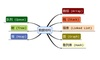数据结构 订阅